## Python 不是 C已翻译 100%

oschina 投递于 2015/07/03 07:40 (共 7 段, 翻译完成于 07-03)

#### 参与翻译 (2人) : gx老苗, 纵横九洲

def closest_distance(lat,lon,trkpts):
d = 100000.0
best = -1
r = trkpts.index
for i in r:
lati = trkpts.ix[i,'Lat']
loni = trkpts.ix[i,'Lon']
md = distance_on_unit_sphere(lat, lon, lati, loni)
if d > md
best = i
d = md
return best

def manhattan_distance(lat1, lon1, lat2, lon2):
lat = (lat1+lat2)/2.0

def manhattan_distance1(lat1, lon1, lat2, lon2):
return abs(lat1-lat2)+abs(lon1-lon2)

closest 函数修改为:

def closest_manhattan_distance1(lat,lon,trkpts):
d = 100000.0
best = -1
r = trkpts.index
for i in r:
lati = trkpts.ix[i,'Lat']
loni = trkpts.ix[i,'Lon']
md = manhattan_distance1(lat, lon, lati, loni)
if d > md
best = i
d = md
return best

def closest_manhattan_distance2(lat,lon,trkpts):
d = 100000.0
best = -1
r = trkpts.index
for i in r:
lati = trkpts.ix[i,'Lat']
loni = trkpts.ix[i,'Lon']
md = abs(lat-lati)+abs(lon-loni)
if d > md
best = i
d = md
return best

def closest(lat,lon,trkpts):
cl = numpy.abs(trkpts.Lat - lat) + numpy.abs(trkpts.Lon - lon)
return cl.idxmin()

Update on July 2, 2015。文章讨论在Hacker News。一些评论没有注意到（missed ）我用到了 pandas 数据帧的情况。主要是它在数据分析中很常用。如果我只是要快速的查询最短距离点，且我时间充分，我可以使用 C 或 C++ 编写四叉树（实现）。

Second update on July 2, 2015。有个评论提到 numba 也能对代码提速。我就试了一下。

`\$ conda install numba`

@jit
def closest_func(lat,lon,trkpts,func):
d = 100000.0
best = -1
r = trkpts.index
for i in r:
lati = trkpts.ix[i,'Lat']
loni = trkpts.ix[i,'Lon']
md = abs(lat - lati) + abs(lon - loni)
if d > md:
#print d, dlat, dlon, lati, loni
best = i
d = md
return best

@jit(nopython=True)
def closest_func(lat,lon,trkpts,func):
d = 100000.0
best = -1
r = trkpts.index
for i in r:
lati = trkpts.ix[i,'Lat']
loni = trkpts.ix[i,'Lon']
md = abs(lat - lati) + abs(lon - loni)
if d > md:
#print d, dlat, dlon, lati, loni
best = i
d = md
return best

### 评论(39)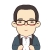#### 引用来自“fireflyc”的评论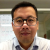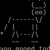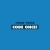#### 引用来自“Xtay”的评论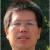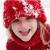#### 引用来自“IdleMan”的评论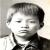#### 引用来自“gzwxn”的评论